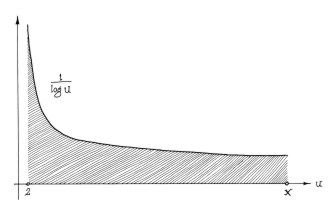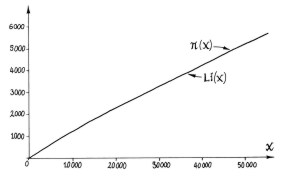The first improvement on x/log x we consider is the logarithmic integral function Li(x). This is defined to be the area under the curve of the function 1/log u between 2 and x, as illustrated in the lefthand figure. Gauss arrived at this from the empirical fact that the probability of finding a prime number at an integer value near a very large number x is almost exactly 1/log x. l'Hopital's rule can be used to show that the ratio of x/log x to Li(x) tends to 1 as x approaches infinity. Thus we may use either expression as an approximation to π(x) in the statement of the prime number theorem. In the righthand figure we see that this function provides an excellent fit to the function π(x). As Zagier states, "within the accuracy of our picture, the two coincide exactly." << previous      beginning      next >>